# G.3.6 Practice Problems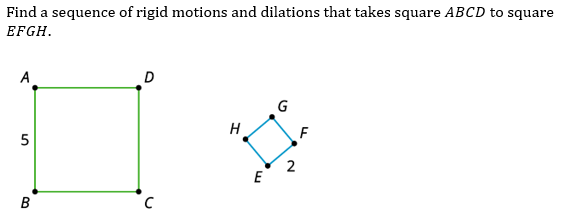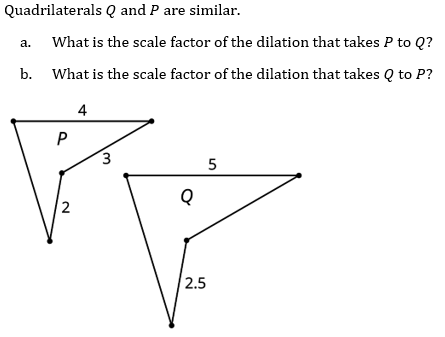What is our definition of similarity?

Select all that apply
• A
• B
• C
• D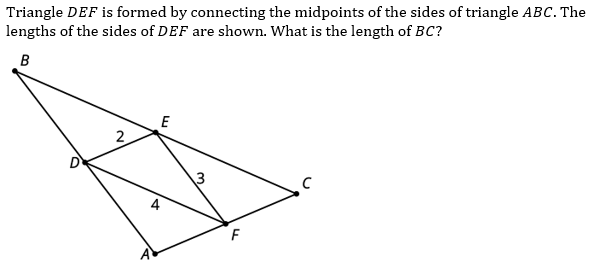Select all that apply
• A
• B
• C
• D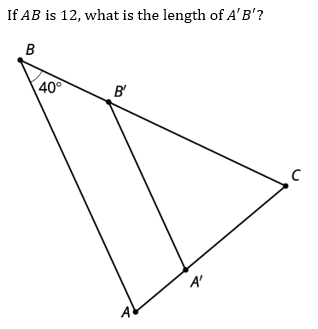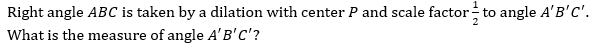A polygon has perimeter 12. It is dilated with a scale factor of k and the resulting image has a perimeter of 8. What is the scale factor?

Select all that apply
• A
• B
• C
• D